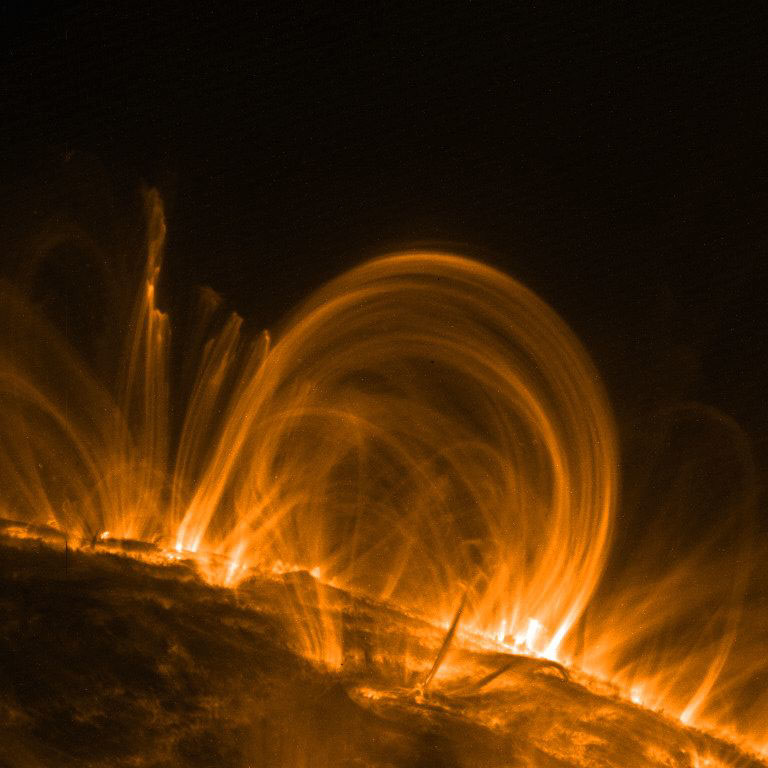# Dimensionless parameters (plasmapy.formulary.dimensionless)

Module of dimensionless plasma parameters.

These are especially important for determining what regime a plasma is in. (e.g., turbulent, quantum, collisional, etc.).

For example, plasmas at high (much larger than 1) Reynolds numbers are highly turbulent, while turbulence is negligible at low Reynolds numbers.

## Functions

 beta(T, n, B) Compute the ratio of thermal pressure to magnetic pressure. Debye_number(T_e, n_e) Return the number of electrons within a sphere with a radius of the Debye length. Hall_parameter(n, T, B, ion, particle[, ...]) Calculate the particle Hall parameter for a plasma. Lundquist_number(L, B, density, sigma[, ...]) Compute the Lundquist number Mag_Reynolds(U, L, sigma) Compute the magnetic Reynolds number quantum_theta(T, n_e) Compare Fermi energy to thermal kinetic energy to check if quantum effects are important. Reynolds_number(rho, U, L, mu) Compute the Reynolds number.

## Aliases

PlasmaPy provides aliases of the most common plasma functionality for user convenience. Aliases in PlasmaPy are denoted with a trailing underscore (e.g., alias_). For further details, please refer to the contributor guide’s section on aliases.

 betaH_(n, T, B, ion, particle[, ...]) Alias to Hall_parameter. nD_(T_e, n_e) Alias to Debye_number. Re_(rho, U, L, mu) Alias to Reynolds_number. Rm_(U, L, sigma) Alias to Mag_Reynolds.

## Examples